# birdcage

Creates birdcage (MRI coil)

## Description

The `birdcage` object creates to create a birdcage MRI coil. This antenna is most commonly used in clinical MRI. The antenna structure consists of two circular coils connected by conductive elements called `rungs`. The number of rungs depends on the size of the coil and is generally an even number.

The coil is operated at 64 MHz or 128 MHz. The birdcage can be loaded/excited to model a highpass or lowpass coil.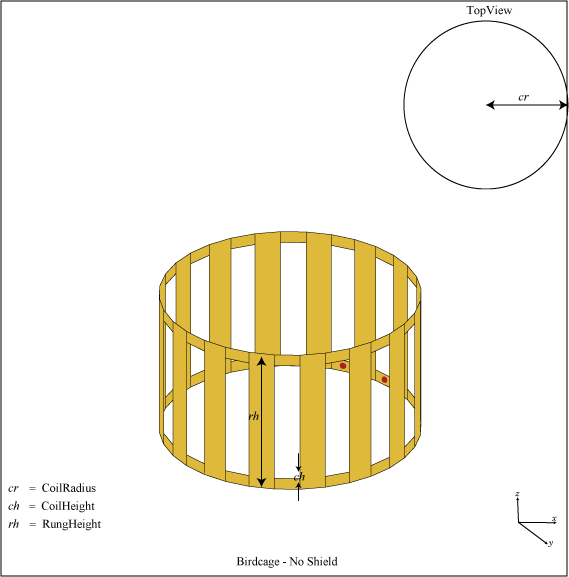## Creation

### Syntax

``bc = birdcage``
``bc = birdcage(Name,Value)``

### Description

example

````bc = birdcage` creates a birdcage antenna to model an MRI coil.```

example

````bc = birdcage(Name,Value)` sets properties using one or more name-value pairs. For example, ```bc = birdcage('NumRungs',8)``` creates a birdcage with eight rungs. Enclose each property name in quotes.```

## Properties

expand all

Number of rungs, specified as a scalar.

Example: `'NumRungs',20`

Example: `bc.NumRungs = 20`

Data Types: `int8`

Coil radius, specified as a scalar in meters.

Example: `'CoilRadius',0.2`

Example: `bc.CoilRadius = 0.2`

Data Types:

Coil height, specified as a scalar in meters.

Example: `'CoilHeight',0.089`

Example: `bc.CoilHeight = 0.089`

Data Types: `double`

Height of rungs, specified as a scalar in meters. Distance is measured from the middle of the upper coil to the middle of the lower coil.

Example: `'RungHeight',0.56`

Example: `bc.RungHeight = 0.56`

Data Types: `double`

Shield radius, specified as a scalar in meters. A value of zero indicates that the shield is absent.

Example: `'ShieldRadius',0.2`

Example: `bc.ShieldRadius = 0.2`

Data Types: `double`

Shield height, specified as a scalar in meters. A value of zero indicates that the shield is absent.

Example: `'ShieldHeight',0.089`

Example: `bc.ShieldHeight = 0.089`

Data Types: `double`

Dielectric mesh to load birdcage, specified as a structure having the following fields:

Points in custom dielectric mesh, specified as an N-by-3 matrix in meters. N is the number of points.

You can use the phantom property to insert a dielectric mesh in the shape of a human head into the bird cage antenna. This dielectric cylinder has a permeability of 80. You can upload this mesh in the form of a mat file.

Data Types: `double`

Tetrahedra in custom dielectric mesh, specified as an M-by-4 integer matrix. M is the number of tetrahedra.

Data Types: `double`

Relative permittivity of dielectric material, specified as a scalar.

Data Types: `double`

Loss in dielectric material, specified as a scalar.

Data Types: `double`

Data Types: `struct`

Location of feeds in Cartesian coordinates, specified as an N-by-3 matrix. You can also use the `getLowPassLocs` and `getHighPassLocs` functions to determine the feed locations in low-pass or high-pass mode.

Example: ```'FeedLocations'= [0.3981 0.0392 -0.2300;0.3528 0.1886 -0.2300]```

Example: ```b.FeedLocations = getLowPassLocs(b)```

Data Types: `double`

Magnitude of voltage applied to each feed, specified as a scalar or 1-by-N vector with each element unit in volts.

Example: `'FeedVoltage',2`

Example: `bc.FeedVoltage = 2`

Data Types: `double`

Phase shift to the excitation voltage at each feed, specified as a scalar or 1-by-M vector with each element unit in degrees.

Example: `'FeedPhase',45`

Example: `bc.FeedPhase = 45`

Data Types: `double`

Type of the metal used as a conductor, specified as a metal material object. You can choose any metal from the `MetalCatalog` or specify a metal of your choice. For more information, see `metal`. For more information on metal conductor meshing, see Meshing.

Example: ```m = metal('Copper'); 'Conductor',m```

Example: ```m = metal('Copper'); ant.Conductor = m```

Lumped elements added to the antenna feed, specified as a lumped element object. You can add a load anywhere on the surface of the antenna. By default, it is at the origin. For more information, see `lumpedElement`.

Example: `'Load',lumpedelement`. `lumpedelement` is the object for the load created using `lumpedElement`.

Example: ```bc.Load = lumpedElement('Impedance',75)```

Tilt angle of the antenna, specified as a scalar or vector with each element unit in degrees. For more information, see Rotate Antennas and Arrays.

Example: `Tilt=90`

Example: `Tilt=[90 90]`,`TiltAxis=[0 1 0;0 1 1]` tilts the antenna at 90 degrees about the two axes defined by the vectors.

Note

The `wireStack` antenna object only accepts the dot method to change its properties.

Data Types: `double`

Tilt axis of the antenna, specified as:

• Three-element vector of Cartesian coordinates in meters. In this case, each coordinate in the vector starts at the origin and lies along the specified points on the X-, Y-, and Z-axes.

• Two points in space, each specified as three-element vectors of Cartesian coordinates. In this case, the antenna rotates around the line joining the two points in space.

• A string input describing simple rotations around one of the principal axes, 'X', 'Y', or 'Z'.

Example: `TiltAxis=[0 1 0]`

Example: `TiltAxis=[0 0 0;0 1 0]`

Example: `TiltAxis = 'Z'`

Data Types: `double`

## Object Functions

 `getLowPassLocs` Feeding location to operate birdcage as lowpass coil `getHighPassLocs` Feeding location to operate birdcage as highpass coil `show` Display antenna, array structures or shapes `axialRatio` Axial ratio of antenna `beamwidth` Beamwidth of antenna `charge` Charge distribution on antenna or array surface `current` Current distribution on antenna or array surface `efficiency` Radiation efficiency of antenna `EHfields` Electric and magnetic fields of antennas; Embedded electric and magnetic fields of antenna element in arrays `impedance` Input impedance of antenna; scan impedance of array `mesh` Mesh properties of metal, dielectric antenna, or array structure `meshconfig` Change mesh mode of antenna structure `optimize` Optimize antenna or array using SADEA optimizer `pattern` Radiation pattern and phase of antenna or array; Embedded pattern of antenna element in array `patternAzimuth` Azimuth pattern of antenna or array `patternElevation` Elevation pattern of antenna or array `rcs` Calculate and plot radar cross section (RCS) of platform, antenna, or array `returnLoss` Return loss of antenna; scan return loss of array `sparameters` Calculate S-parameter for antenna and antenna array objects `vswr` Voltage standing wave ratio of antenna

## Examples

collapse all

Create and view a default birdcage antenna.

`bc = birdcage`
```bc = birdcage with properties: NumRungs: 16 CoilRadius: 0.4000 CoilHeight: 0.0400 RungHeight: 0.4600 ShieldRadius: 0 ShieldHeight: 0 Phantom: [] FeedLocations: [2x3 double] FeedVoltage: 1 FeedPhase: 0 Conductor: [1x1 metal] Tilt: 0 TiltAxis: [1 0 0] Load: [1x1 lumpedElement] ```
`show(bc);`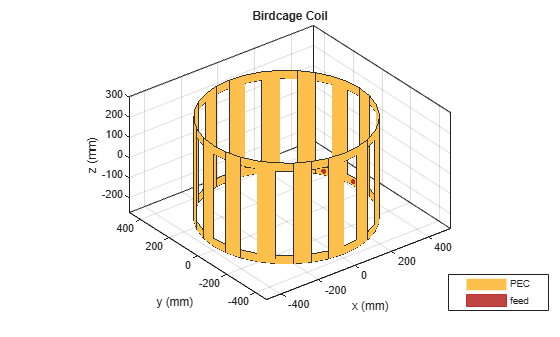Plot the radiation pattern at 128 MHz.

`pattern(bc,128e6)`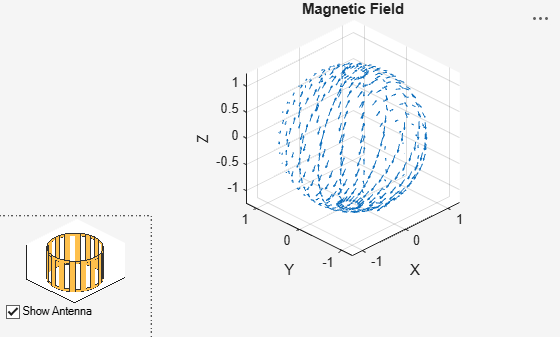Antenna Toolbox™ provides two `.mat` files to load a phantom human head model into a birdcage antenna. The humanheadcoarse.mat contains a coarse dielectric mesh of the human head model and the humanheadfine.mat provides the user with a finer dielectric mesh. Load the coarse human head model.

Load human head model file. Extract the values of `Points` and `Tetrahedra`. Add a relative permittivity (EpsilonR) of 10 and a dielectric loss (LossTangent) of 0.002. Scale the dielectric mesh to fit in the birdcage antenna. In this case, the mesh points are multiplied by 0.003.

```load humanheadcoarse.mat humanhead = struct('Points',0.003*P,'Tetrahedra',T,'EpsilonR',10,... 'LossTangent',0.002)```
```humanhead = struct with fields: Points: [584x3 double] Tetrahedra: [2818x4 double] EpsilonR: 10 LossTangent: 0.0020 ```

`b = birdcage('Phantom',humanhead)`
```b = birdcage with properties: NumRungs: 16 CoilRadius: 0.4000 CoilHeight: 0.0400 RungHeight: 0.4600 ShieldRadius: 0 ShieldHeight: 0 Phantom: [1x1 struct] FeedLocations: [2x3 double] FeedVoltage: 1 FeedPhase: 0 Conductor: [1x1 metal] Tilt: 0 TiltAxis: [1 0 0] Load: [1x1 lumpedElement] ```
`show(b)`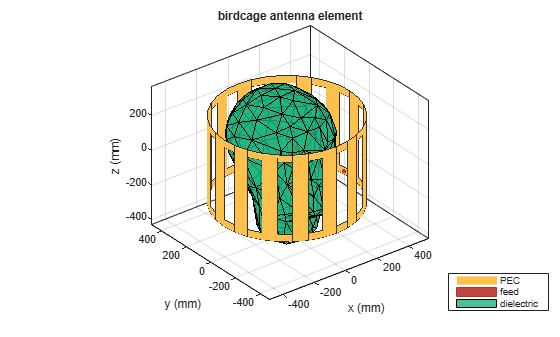Create a birdcage antenna.

```b = birdcage; show(b);```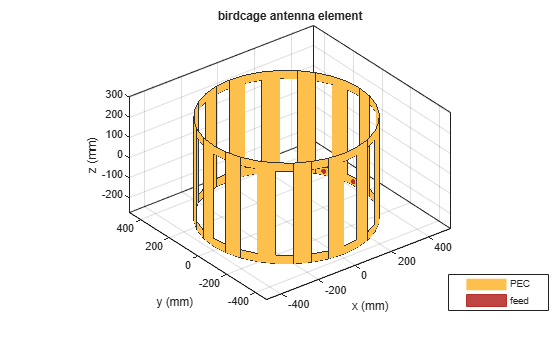Use the birdcage as a high-pass coil.

`b.FeedLocations = getHighPassLocs(b)`
```b = birdcage with properties: NumRungs: 16 CoilRadius: 0.4000 CoilHeight: 0.0400 RungHeight: 0.4600 ShieldRadius: 0 ShieldHeight: 0 Phantom: [] FeedLocations: [32x3 double] FeedVoltage: 1 FeedPhase: 0 Conductor: [1x1 metal] Tilt: 0 TiltAxis: [1 0 0] Load: [1x1 lumpedElement] ```
`show(b);`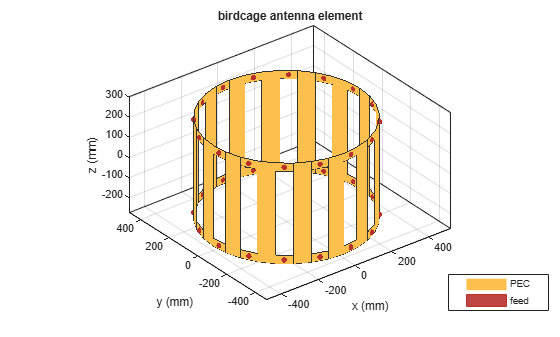Shield the antenna to ensure that radiation does not leak out.

```b.ShieldRadius = 0.5; b.ShieldHeight = 0.5; show(b) ;```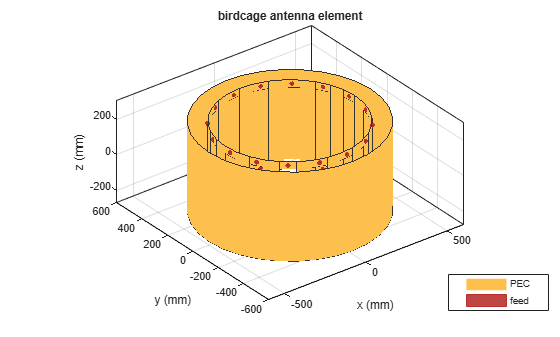## Version History

Introduced in R2017b